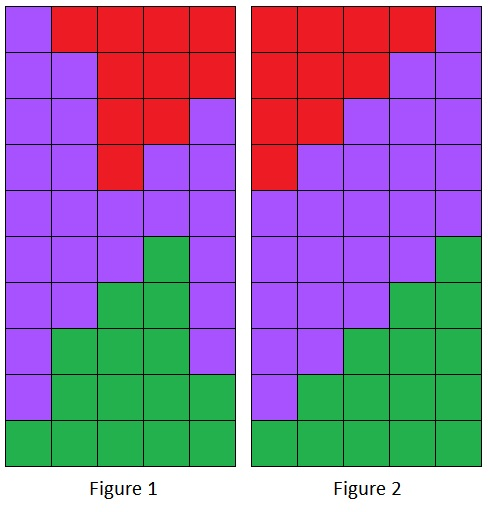# A Geometric Proof of Proizvolov's Identity

Stuart Anderson

As in the description at another page consider the numbers A = \{a_1,\cdots,a_N\} chosen from S = \{1,\cdots, 2N\}, and the set complement B = S\setminus A = \{b_1,\cdots,b_N\}. Let the a_i be arranged in ascending order and the b_i in descending order. Proizvolov's identity says that

\sum |a_i - b_i| = N^2\;.

On a coordinate system, let y_1=a_i for i-1 \le x \lt i be a staircase function and y_2=b_i for i-1 \le x \lt i be another staircase function. Enclose the graphs of the two functions inside the rectangle 0 \le x \lt N,\;0 \le y \lt 2N. Then the sum above is the (unsigned) area between the graphs of y_1 and y_2, shown in light blue in Figure 1.However, this area is the same as the area of the rectangle minus the area above both graphs (red in Figure 1) and the area below both graphs (green in Figure 1). Consider these areas instead. They are defined by the staircase functions y_3=c_i for i-1 \le x \lt i and y_4=d_i for i-1 \le x \lt i, where c_i = \max(a_i,\; b_i) and d_i = \min(a_i,\; b_i). It is visually obvious that every point on the graph of y_3 lies above the entirety of the graph of y_4; hence the values c_i must be exactly the numbers N+1,\cdots,\; 2N (by the pigeonhole principle), and therefore d_i must be the numbers 1,\cdots,\;N.

Now the area above y_3 consists of a sum of rectangles, and it will not change if these rectangles are rearranged, and similarly for the area below y_4. Therefore, arrange both the sets \{c_i\} and \{d_i\} values into ascending order and replot the graphs that result (Figure 2).

Since the area above and below them has not changed, neither has the area between them. But it now consists of exactly N rectangles each of height N, and so the area is N^2. This completes the proof.